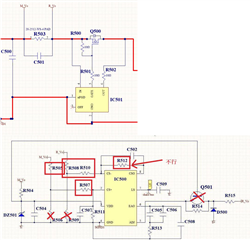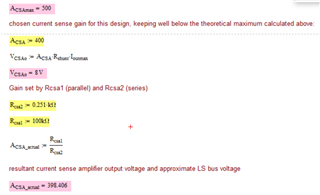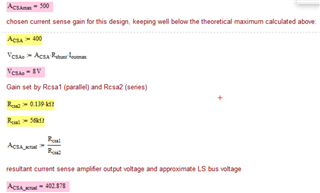If you have a related question, please click the "Ask a related question" button in the top right corner. The newly created question will be automatically linked to this question.

Part Number: UCC29002

Hi Team

There is a good new that we DIN UCC29002

And there have one thing need your support!

Cause need LS voltage is 8V,
From the calculation formula below, I found out why R512 value cannot use 100K
But LS voltage can be 8V when R512= 56K, why? THX

R505,R508 51 ohm

R507,R510 200ohm

R512 100k

R503 0.002 ohm

Io=10A

Acas=R512/(R508+R510)=100k/0.251k=398.4

LS=0.002*10*398.4=7.968V

R505,R508 39 ohm

R507,R510 100ohm

R512 56k

R503 0.002 ohm

Io=10A

Acas=R512/(R508+R510)=56k/0.139k=402.87

LS=0.002*10*402.87=8.05V• Hi Cheng,

thanks for your interest in UCC29002.

both of your two kind of parameters are correct and can be used.for first parameter